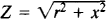# Impedance and Resistance

The following article is from The Great Soviet Encyclopedia (1979). It might be outdated or ideologically biased.

## Impedance and Resistance

(also electrical impedance and electrical resistance; in Russian, elektricheskoe soprotivlenie). Impedance is a quantity characterizing the total opposition presented to an alternating current by a circuit or part of a circuit. Resistance is a quantity characterizing the opposition offered to the flow of direct current by a circuit or part of a circuit; in AC circuits, resistance is the real part of impedance. Both impedance and resistance are measured in ohms (Ω).

Opposition to the flow of a current consists in either the transfer of electric energy or the conversion of electric energy into other forms of energy. In the case of an alternating current, the opposition that consists in the irreversible conversion of electric energy into other forms of energy, primarily heat, is called resistance ; the opposition that consists in the transfer of electric energy to or from the electric or magnetic field produced is known as reactance.

In the case of direct current, the resistance R of a circuit is equal, in accordance with Ohm’s law, to the quotient of the voltage U applied to the circuit and the current I that flows through the circuit in the absence of other current sources or an electromotive force in the circuit.

In the case of a sinusoidal current, the impedance of a circuit is equal towhere r is the circuit’s resistance and x is its reactance. Reactance is established by the presence of inductance and capacitance in a circuit (seeREACTANCE, INDUCTIVE and REACTANCE, CAPACITIVE).

The resistance of a circuit element depends both on the shape and size of the element and on the material of which the element is made. For an element of homogeneous composition in the form of a bar, plate, or wire of constant cross section S and length l, R = ρ × (l/S), where ρ is the resistivity, or specific resistance. Resistivity characterizes the material of which a circuit element is made; it is measured in ohm-meters (Ω · m), in ohm-centimeters (Ω·cm), or in Ω·mm2/m. According to their resistivity, all substances are divided into conductors (see and ), semiconductors (seeSEMICONDUCTOR and SEMICONDUCTOR MATERIAL), and insulators (seeDIELECTRICS and INSULATING MATERIAL, ELECTRICAL). At very low temperatures, the resistance of certain metals and alloys drops to zero (seeSUPERCONDUCTIVITY and SUPERCONDUCTOR).

Conductivity, a quantity that is the reciprocal of resistivity, is often introduced as an alternative to resistivity, especially in cases where the physical nature of resistance is considered.

The term “resistance” is commonly used in relation to a resistor or some other circuit element that is connected to a circuit in order, for example, to limit or control the current flowing through the circuit (seeSHUNT, RHEOSTAT, and POTENTIOMETER).